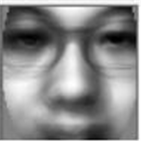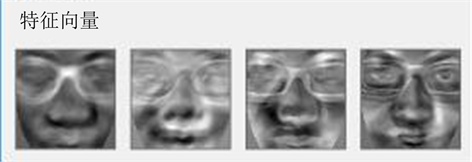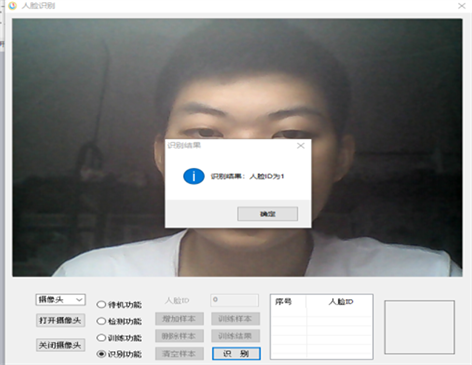﻿ 基于特征脸的人脸识别研究

# 基于特征脸的人脸识别研究Face Recognition Based on Feature Faces

Abstract: Face recognition is a technology to recognize faces by analyzing facial and facial features. In this pa-per, a face recognition method based on PCA is described, and image preprocessing technology is applied on the basis of this algorithm, and then the recognition simulation experiment is carried out. This method recognizes and classifies human faces by comparing the spatial distance between the face image and the trained feature face. The experiment validates the feature face algorithm successfully.

1. 前言

2. 图像预处理

2.1. 图像灰度变换

2.2. 中值滤波

2.3. 直方图均衡化

3. 特征脸方法的原理

3.1. 特征提取

$\psi =\frac{1}{M}\underset{n=1}{\overset{M}{\sum }}{T}_{n}$

${\Phi }_{i}={T}_{i}-\psi$

$C=\underset{n=1}{\overset{M}{\sum }}{\Phi }_{n}{\Phi }_{n}^{\text{T}}=A{A}^{\text{T}}$

$A{A}^{\text{T}}\left(A{V}_{i}\right)={\mu }_{i}\left(A{V}_{i}\right)$

3.2. 根据特征子空间进行识别

${w}_{i}={U}^{\text{T}}\left({T}_{i}-\psi \right)$

${\theta }_{C}=\frac{1}{2}\underset{m,n}{\mathrm{max}}\left\{||{w}_{m}-{w}_{n}||\right\}\text{\hspace{0.17em}}\text{\hspace{0.17em}}\text{\hspace{0.17em}}\left(m,n=1,\cdots ,M\right)$

$w={U}^{\text{T}}\left(T-\psi \right)$

4. 实验结果Figure 1. Average faceFigure 2. EigenvectorFigure 3. Face recognition effect

5. 总结

NOTES

*通讯作者

 何荣. 基于OpenCV的人脸识别系统设计[D]: [硕士学位论文]. 广东: 华南理工大学, 2013.

 沈慧钧. 关于人脸识别图像预处理方法的研究与实现[J]. 科技与创新, 2014(18): 119-120.

 张宁. 基于PCA算法的人脸识别研究[J]. 山西电子技术, 2009(2): 23-24.

 邢志恒. 基于特征脸的人脸识别系统研究[J]. 电脑知识与技术, 2007(9): 1431.

 闫宏, 张兴周, 刘晓瑞. 基于特征脸的人脸识别系统[J]. 应用技术, 2007(4): 20-23.

 孔令钊, 唐文静. 基于PCA的人脸识别系统研究与实现[J]. 计算机仿真, 2012(6): 27-29.

Top